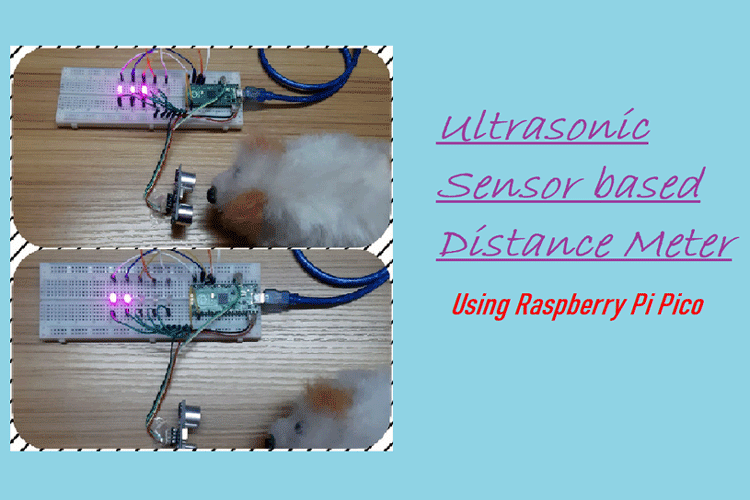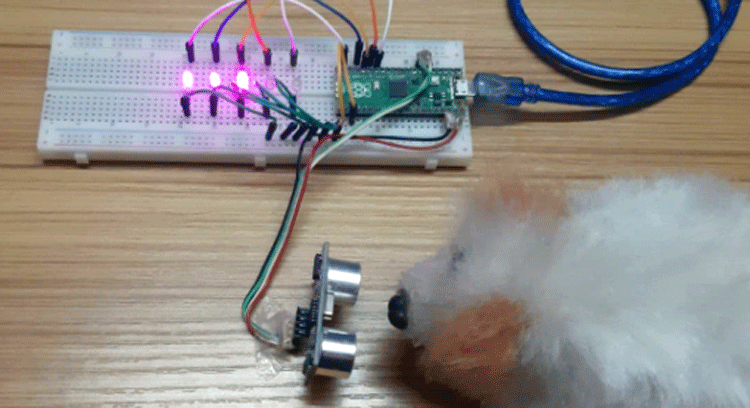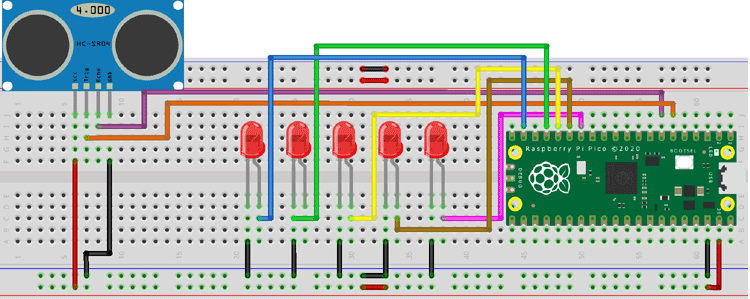1380 31
Innovation XYZ

## Raspberry Pi Pico based Distance Meter

The distance meter is used for accurately determining the distance of an object without contact by...

##### Description:-

The distance meter is used for accurately determining the distance of an object without contact by the medium of ultrasonic sound waves(20,000 Hz above sound frequency). This project will become very important instrument in practical if it is used perfectly. If case we use a laser range finder it will not be efficient enough since laser beams will pass through transparent medium(object) that will not be able to detect it. So this is a way more efficient. Also, this project can be used in cars so as to detect any obstacle while parking. Or check the distance left to park safe fully.### Project Used Hardware

Raspberry Pi Pico, Ultrasonic sensor, LEDs, Breadboard, Jumper wires

Thonny IDE

### Project Hardware Software Selection

I have used the Raspberry Pi Pico because it is a very small, low cost microcontroller board. Also this board is much faster than any other small microcontroller boards like Arduino Nano, or Arduino Pro Mini since it has a clock frequency of 133 MHz I have also chosen the ultrasonic sensor because it is more efficient in comparison to Laser Finder. LED to detect the approximate distance. I have used Thonny IDE to program the Raspberry Pi Pico using (Micro Python). Since Micro Python requires very small codes in comparison to Arduino IDE.

### Circuit DiagramHere in the Pico board, I have reserved 5 GPIO pins for 5 LEDs, 2 GPIO pins for the Trigger and Echo pin of the ultrasonic sensor. GPIO pin 6 and 7 are for the Trigger and Echo pins, respectively. GPIO pin 10, 11, 12, 13 and 14 are for the LEDs. The negative terminal of all LEDs and the ultrasonic sensor are connected together with the Ground pin of the Pico and the VCC pin of the sensor is connected to 5v of the Pico. The LEDs from GPIO pin 10 to 14 will start to glow gradually as the distance between the object and the sensor decreases(i.e. when the object come closer).

##### Code

``` ```

``````# Ultrasonic Distance Meter using Raspberry Pi Pico
#Micro Python
from machine import Pin,PWM #importing PIN and PWM
import time #importing time
import utime
#Defining LED pins
LED1=Pin(10,Pin.OUT)
LED2=Pin(11,Pin.OUT)
LED3=Pin(12,Pin.OUT)
LED4=Pin(13,Pin.OUT)
LED5=Pin(14,Pin.OUT)
#Defining  Trigger and Echo pins
trigger = Pin(4, Pin.OUT)
echo = Pin(5, Pin.IN)
# Defining function to get distance from ultrasonic sensor
def get_distance():
trigger.low()
utime.sleep_us(2)
trigger.high()
utime.sleep_us(5)
trigger.low()
while echo.value() == 0:
signaloff = utime.ticks_us()
while echo.value() == 1:
signalon = utime.ticks_us()
timepassed = signalon - signaloff
dist = (timepassed * 0.0343) / 2
return dist
while True:
distance=get_distance() #Getting distance in cm
print(+distance)
#Defining LED ON and OFF based on conditions
if distance <=3:  #If distance is less or equal to 3cm
LED1.high()
LED2.high()
LED3.high()
LED4.high()
LED5.high()
elif distance <=5:  #If distance is less or equal to 5cm but greater than 3cm
LED1.low()
LED2.high()
LED3.high()
LED4.high()
LED5.high()
elif distance <=7:  #If distance is less or equal to 7cm but greater than 5cm
LED1.low()
LED2.low()
LED3.high()
LED4.high()
LED5.high()
elif distance <=10:  #If distance is less or equal to 10cm but greater than 7cm
LED1.low()
LED2.low()
LED3.low()
LED4.high()
LED5.high()
elif distance ==15: #If distance is equal to 15cm
LED1.low()
LED2.low()
LED3.low()
LED4.low()
LED5.high()
elif distance >15: #If distance is greater than 15cm
LED1.low()
LED2.low()
LED3.low()
LED4.low()
LED5.low()``````
``` ```